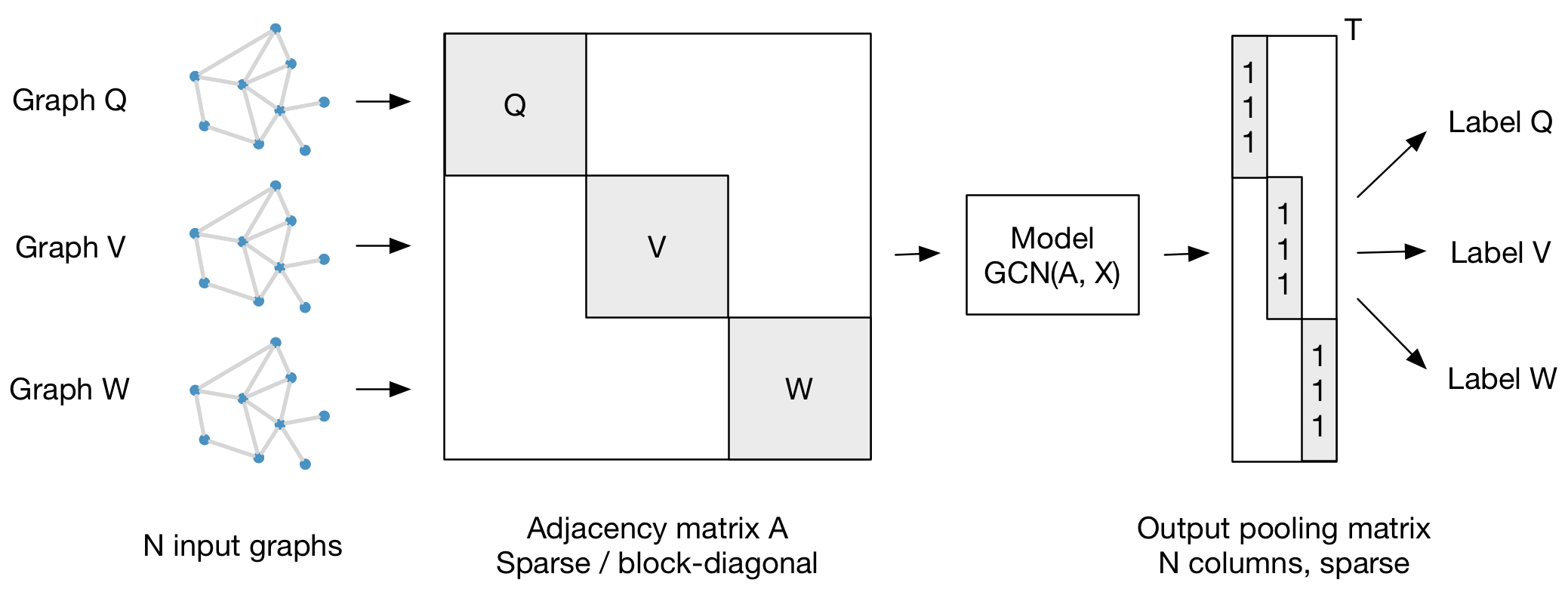### gcn

#### by tkipf

tkipf /gcnImplementation of Graph Convolutional Networks in TensorFlow

Available items#### No Items, yet!

The developer of this repository has not created any items for sale yet. Need a bug fixed? Help with integration? A different license? Create a request here:

# Graph Convolutional Networks

This is a TensorFlow implementation of Graph Convolutional Networks for the task of (semi-supervised) classification of nodes in a graph, as described in our paper:

Thomas N. Kipf, Max Welling, Semi-Supervised Classification with Graph Convolutional Networks (ICLR 2017)

For a high-level explanation, have a look at our blog post:

Thomas Kipf, Graph Convolutional Networks (2016)

## Installation

```python setup.py install
```

## Requirements

• tensorflow (>0.12)
• networkx

## Run the demo

```cd gcn
python train.py
```

## Data

In order to use your own data, you have to provide * an N by N adjacency matrix (N is the number of nodes), * an N by D feature matrix (D is the number of features per node), and * an N by E binary label matrix (E is the number of classes).

Have a look at the

`load_data()`
function in
`utils.py`
for an example.

In this example, we load citation network data (Cora, Citeseer or Pubmed). The original datasets can be found here: http://www.cs.umd.edu/~sen/lbc-proj/LBC.html. In our version (see

`data`
folder) we use dataset splits provided by https://github.com/kimiyoung/planetoid (Zhilin Yang, William W. Cohen, Ruslan Salakhutdinov, Revisiting Semi-Supervised Learning with Graph Embeddings, ICML 2016).

You can specify a dataset as follows:

```python train.py --dataset citeseer
```

(or by editing

`train.py`
)

## Models

You can choose between the following models: *

`gcn`
: Graph convolutional network (Thomas N. Kipf, Max Welling, Semi-Supervised Classification with Graph Convolutional Networks, 2016) *
`gcn_cheby`
: Chebyshev polynomial version of graph convolutional network as described in (Michaël Defferrard, Xavier Bresson, Pierre Vandergheynst, Convolutional Neural Networks on Graphs with Fast Localized Spectral Filtering, NIPS 2016) *
`dense`
: Basic multi-layer perceptron that supports sparse inputs

## Graph classification

Our framework also supports batch-wise classification of multiple graph instances (of potentially different size) with an adjacency matrix each. It is best to concatenate respective feature matrices and build a (sparse) block-diagonal matrix where each block corresponds to the adjacency matrix of one graph instance. For pooling (in case of graph-level outputs as opposed to node-level outputs) it is best to specify a simple pooling matrix that collects features from their respective graph instances, as illustrated below:## Cite

Please cite our paper if you use this code in your own work:

```@inproceedings{kipf2017semi,
title={Semi-Supervised Classification with Graph Convolutional Networks},
author={Kipf, Thomas N. and Welling, Max},
booktitle={International Conference on Learning Representations (ICLR)},
year={2017}
}
```